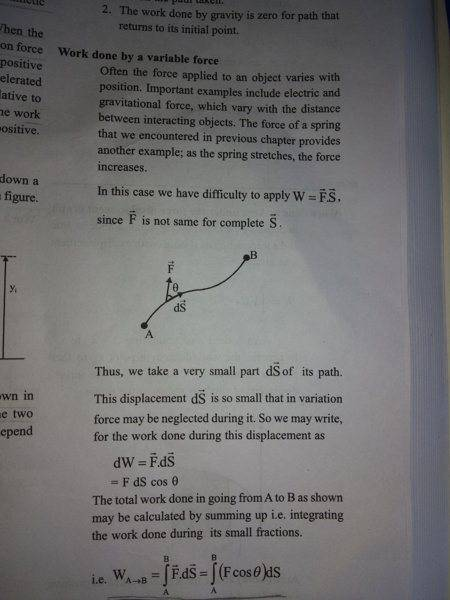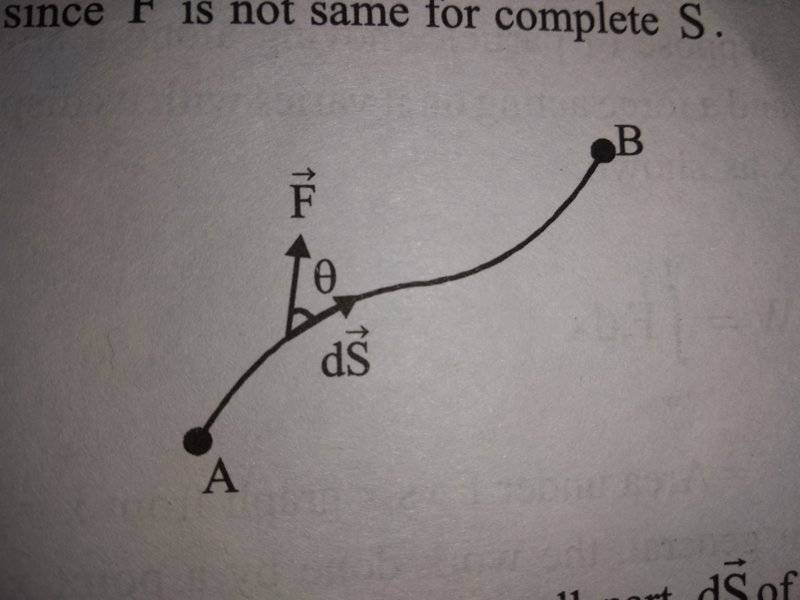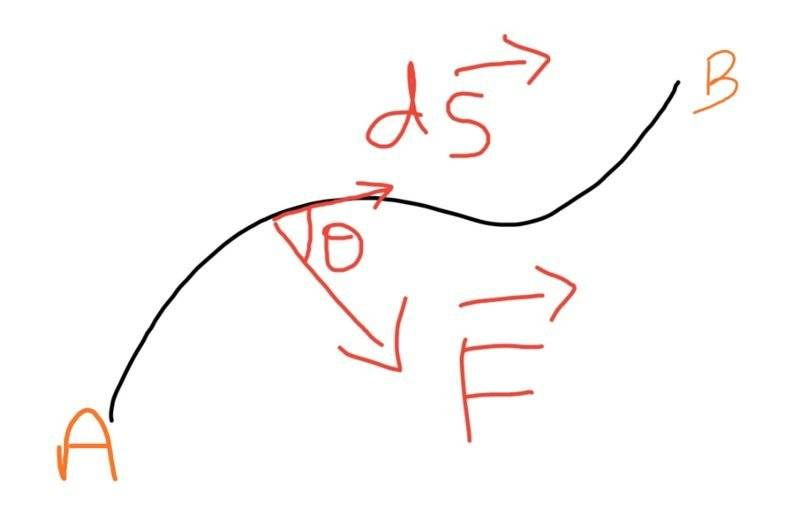# Calculation of work done by a variable force

• navneet9431

Gold Member

## Homework Statement

See the explanation of work done by variable forces given in my textbook.For calculation of work done by variable forces the author analyses only a small fraction of displacement "ds".
And then he makes **assumption** that the force is acting on the particle at an angle theta and that too in the *upward* direction.And then he integrates them.
My question is how integrating them would give us the total work done by the variable force.Because at different positions the angle at which the force is acting would change.It would not be 'theta' for all instants.
And my next question is that how should we determine in which direction the force is acting?
Like in my book the author assumed that it would act like this(in the upward direction),So what if I say that the force would not act like that but would act like this in the downward direction?W=Fcos(theta)*S

## The Attempt at a Solution

I think that the direction of force should be determined by the curve if the path.If the path bends inwards then the force would act downwards and if the path is bending upwards then the force would act upwards.
Is my thinking correct?
I will be thankful for any help!

#### Attachments

at different positions the angle at which the force is acting would change
The author allows that θ is a variable.

Ok then integrating them would be wrong as the angle would be changing continuously at every instant.
So integrating them would not give us the actual work done,but slightly different value.
Am I correct?
The author allows that θ is a variable.

then integrating them would be wrong
No, integrating is fine as long as it is treated as a variable. The mistake would be to move the cos(θ) term outside of the integral.

•navneet9431
No, integrating is fine as long as it is treated as a variable. The mistake would be to move the cos(θ) term outside of the integral.
Thanks!
Now check my this reasoning,is it correct?

"I think that the direction of force should be determined by the curve of the path.If the path bends inwards then the force would act downwards and if the path is bending upwards then the force would act upwards."

the direction of force should be determined by the curve if [of] the path
The author is not assuming the force is constant either, neither in magnitude nor direction.

"I think that the direction of force should be determined by the curve of the path.If the path bends inwards then the force would act downwards and if the path is bending upwards then the force would act upwards."
You are assuming that this force is the only force acting on the object. This is not necessarily true.

A representative diagram showing a force in a direction does not mean that the force is always in that direction, or has that magnitude. In general, both the magnitude and direction (θ) are variables and are part of the integrand. Note also that ds itself changes as you follow the path. Also note post #7 above, There could be three different forces acting on the object in different directions, and you may need to calculate the work done by one of the forces.

The author is assuming you already know (in advance) the magnitude and direction of the force along the path, in terms of their variation with path position s.